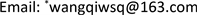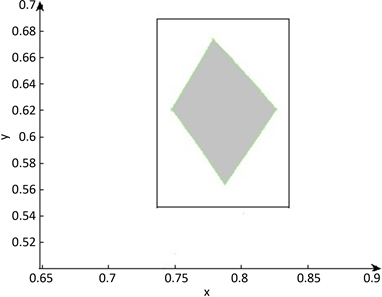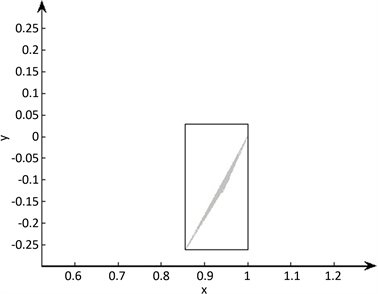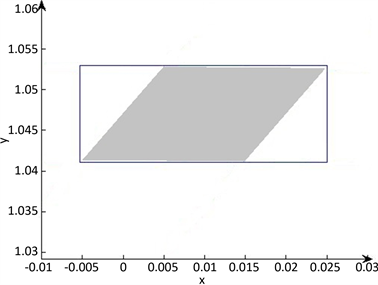﻿ 求解区间参数非线性方程组的数值方法 Numerical Method for Solving Nonlinear Equations with Interval Parameter

Vol.06 No.07(2017), Article ID:22546,10 pages
10.12677/AAM.2017.67105

Numerical Method for Solving Nonlinear Equations with Interval Parameter

Qi Wang, Wang Xiao, Haijun Wang*

School of Mathematics, China University of Mining and Technology, Xuzhou JiangsuReceived: Oct. 8th, 2017; accepted: Oct. 24th, 2017; published: Oct. 31st, 2017ABSTRACT

In this paper, the problem of solving nonlinear equations with interval parameters is considered. By improving interval Krawczyk operator and applying Newton order-reduction method, we propose an interval algorithm to solve nonlinear equations with interval parameters. The theoretical result and numerical test are given and numerical result shows that proposed interval algorithm can effectively solve nonlinear equations with interval parameters.

Keywords:Nonlinear Equations with Interval Parameters, Newton Order-Reduction Method, Krawczyk Operator1. 引言

$f\left(x,\left[p\right]\right)=0,$ (1)

$f\left(x\right)=0,$ (2)

$\sum =\left\{x\in {R}^{n}:\exists f\left(x\right)\in f\left(x\right)\text{}s.t.\text{}f\left(x\right)=0\right\}.$

1966年Moore在  《Interval Analysis》一书中第一次用区间Newton法逼近了一个简单的区间方程的零解；1969年Krawczyk  针对Moore的区间Newton程序在计算量方面的缺陷(求区间矩阵的逆计算量比较大)提出了区间Krawczyk算子；1992年Hansen  利用牛顿法提出了区间搜索和启发式的终止条件求解区间方程的零解；2006年Nikas  等在理论上提出了一种边界近似的方法求解区间方程，并取得了很好的成果；2009年Nikas  等又基于牛顿法提出了通过分离零解区间端点的方法求解区间方程。2017年邱亮  等基于单调分割技术拓展了Nikas的方法，在计算效率上有所提高。

2. 区间Krawczyk算子与区间扩展

1996年Moore  基于点Newton法提出了区间Newton法，对于非区间方程组 $f\left(x\right)=0$$x\in \left[x\right]\subseteq IR$${x}^{\ast }$ 是方程的解，且 ${x}^{\ast }\subseteq \left[x\right]$ ，则传统的区间牛顿法公式为：

$N\left(\left[x\right]\right)=:m-\frac{F\left(x\right)}{{F}^{\prime }\left(\left[x\right]\right)},$

1969年Krawczyk  推广了Moore的区间Newton法得到了区间Krawczyk算子，

$K\left(\left[x\right]\right)=:m-Yf\left(m\right)+\left(I-Y{F}^{\prime }\left(\left[x\right]\right)\right)\left(\left[x\right]-m\right)$

${F}^{\prime }\left(\left[x\right]\right)=\left[\underset{_}{l},\stackrel{¯}{l}\right],i=1,2,\cdots ,n$ ，点向量 $\underset{_}{c}$$\stackrel{¯}{c}$ 定义为：

${\underset{_}{c}}_{i}=\left\{\begin{array}{l}{\stackrel{¯}{x}}_{i},\text{}{\stackrel{¯}{l}}_{i}\le 0,\\ {\underset{_}{x}}_{i},\text{}{\underset{_}{l}}_{i}\ge 0,\\ \left({\stackrel{¯}{l}}_{i}{\underset{_}{x}}_{i}-{\underset{_}{l}}_{i}{\stackrel{¯}{x}}_{i}\right)/\left({\stackrel{¯}{l}}_{i}-{\underset{_}{l}}_{i}\right),\text{}其他情形,\end{array}$ (3)

${\underset{_}{c}}_{i}=\left\{\begin{array}{l}{\underset{_}{x}}_{i},\text{}{\stackrel{¯}{l}}_{i}\le 0,\\ {\stackrel{¯}{x}}_{i},\text{}{\underset{_}{l}}_{i}\ge 0,\\ \left({\underset{_}{l}}_{i}{\underset{_}{x}}_{i}-{\stackrel{¯}{l}}_{i}{\stackrel{¯}{x}}_{i}\right)/\left({\underset{_}{l}}_{i}-{\stackrel{¯}{l}}_{i}\right),\text{}其他情形,\end{array}$ (4)

$\mathrm{inf}{F}_{M}\left(\left[x\right],c\right)\le \mathrm{inf}{F}_{M}\left(\left[x\right],\underset{_}{c}\right),\mathrm{sup}{F}_{M}\left(\left[x\right],\stackrel{¯}{c}\right)\le \mathrm{sup}{F}_{M}\left(\left[x\right],c\right).$

$\begin{array}{c}{F}_{o}\left(\left[x\right],\stackrel{¯}{c},\underset{_}{c}\right)=\left[\mathrm{inf}{F}_{M}\left(\left[x\right],\underset{_}{c}\right),\mathrm{sup}{F}_{M}\left(\left[x\right],\stackrel{¯}{c}\right)\right]\\ =\left[\mathrm{inf}\left\{f\left(\underset{_}{c}\right)+{F}^{\prime }\left(\left[x\right]\right)\left(\left[x\right]-\underset{_}{c}\right)\right\},\mathrm{sup}\left\{f\left(\stackrel{¯}{c}\right)+{F}^{\prime }\left(\left[x\right]\right)\left(\left[x\right]-\stackrel{¯}{c}\right)\right\}\right].\end{array}$

$\left\{f\left(x\right):x\in \left[x\right]\right\}\subseteq {F}_{o}\left(\left[x\right],\stackrel{¯}{c},\underset{_}{c}\right)\subseteq {F}_{M}\left(\left[x\right],c\right),$ (5)

${F}_{N}\left(\left[x\right]\right)\subseteq {F}_{o}\left(\left[x\right],\stackrel{¯}{c},\underset{_}{c}\right),$

$f\left(x\right)=\left\{\begin{array}{l}{x}_{1}^{3}+\left[0.9,1.1\right]{x}_{2}^{2}-5,\\ \left({x}_{1}+1\right){x}_{2}-\left(3{x}_{1}+\left[0.9,1.1\right]\right).\end{array}$

3. 改进的区间Krawczyk算子

$x=p\left(x\right)=x-Cf\left(x\right),$

$K\left(\left[x\right],c\right)=c-Cf\left(c\right)+\left(I-C{F}^{\prime }\left(\left[x\right]\right)\right)\left(\left[x\right]-c\right)$

1) 如果 ${x}^{\ast }\in \left[x\right]$ ，则 ${x}^{\ast }\in K\left(\left[x\right],c\right)$

2) 如果 $K\left(\left[x\right],c\right)\cap \left[x\right]=\varnothing$ ，则区间 $\left[x\right]$ 不含有区间参数非线性方程组(2)的任何解；

3) 如果 $K\left(\left[x\right],c\right)\subseteq \left[x\right]$ ，则 $\left[x\right]$ 一定包含区间参数非线性方程组(2)的一个解。Table 1. The comparison for effectiveness of interval extension

1) 由于 ${x}^{\ast }$ 为区间参数非线性方程组(2)的一个解，则存在 $f\left(x\right)\in f\left(x\right)$ 使得 $f\left(x\right)\in f\left(x\right)$ ，故

$\begin{array}{c}{x}^{\ast }={x}^{\ast }-Cf\left({x}^{\ast }\right)=c-Cf\left(c\right)+{x}^{\ast }-c-C\left(f\left({x}^{\ast }\right)-f\left(c\right)\right)\\ =c-Cf\left(c\right)+{x}^{\ast }-c-C{f}^{\prime }\left(c+\theta \left({x}^{\ast }-c\right)\right)\left({x}^{\ast }-c\right)\\ =c-Cf\left(c\right)+\left(I-C{f}^{\prime }\left(c+\theta \left({x}^{\ast }-c\right)\right)\right)\left({x}^{\ast }-c\right)\\ \in c-Cf\left(c\right)+\left(I-C{F}^{\prime }\left(\left[x\right]\right)\right)\left(\left[x\right]-c\right)\subseteq K\left(\left[x\right],c\right).\end{array}$

2) 假设 ${x}^{\ast }\in \left[x\right]$ 为区间参数非线性方程组(2)的一个解，由(1)可知 ${x}^{\ast }\in K\left(\left[x\right],c\right)$ 这与条件相矛盾。所以 $\left[x\right]$ 不包含区间参数非线性方程组(2)的解。

3) 对于任意的 $f\left(x\right)\in f\left(x\right)$ ，定义 ${p}_{1}\left(x\right)=x-Cf\left(x\right)$ ，则

$\begin{array}{c}{p}_{1}\left(x\right)=c-Cf\left(c\right)+x-c-C\left(f\left(x\right)-f\left(c\right)\right)=c-Cf\left(c\right)+x-c-C{f}^{\prime }\left(c+\theta \left(x-c\right)\right)\left(x-c\right)\\ =c-Cf\left(c\right)+\left(I-Cf\text{'}\left(c+\theta \left(x-c\right)\right)\right)\left(x-c\right).\end{array}$

${p}_{1}\left(x\right)\in c-Cf\left(c\right)+\left(I-C{F}^{\prime }\left(\left[x\right]\right)\right)\left(x-c\right)\subseteq K\left(\left[x\right],c\right)$ .

$K\left(\left[x\right],\underset{_}{c},\stackrel{¯}{c}\right)=\left[x\right]-CF\left(\left[x\right],\underset{_}{c},\stackrel{¯}{c}\right)=\left[x\right]-C\left({F}_{N}\left(\left[x\right]\right)\right)\cap {F}_{o}\left(\left[x\right],\stackrel{¯}{c},\underset{_}{c}\right)=\left[x\right]-C\left({F}_{N}\left[x\right]\right)\cap {F}_{M}\left(\left[x\right],\underset{_}{c}\right)\cap {F}_{M}\left(\left[x\right],\stackrel{¯}{c}\right)$

1) $K\left(\left[x\right],c\right)\supseteq K\left(\left[x\right],\underset{_}{c},\stackrel{¯}{c}\right)$

2) 如果 ${x}^{\ast }\in \left[x\right]$ ，则 $K\left(\left[x\right],\underset{_}{c},\stackrel{¯}{c}\right)$

3) 如果 $K\left(\left[x\right],\underset{_}{c},\stackrel{¯}{c}\right)\cap \left[x\right]=\varnothing$ ，则区间 $\left[x\right]$ 内不含有区间参数非线性方程组(2)的任何解；

4) 如果 $K\left(\left[x\right],\underset{_}{c},\stackrel{¯}{c}\right)\subseteq \left[x\right]$ ，则 $\left[x\right]$ 一定包含区间参数非线性方程组(2)的一个解。

1) 因为

$K\left(\left[x\right],c\right)=c-Cf\left(c\right)+\left(I-C{F}^{\prime }\left(\left[x\right]\right)\right)\left(\left[x\right]-c\right)=\left[x\right]-C\left(f\left(c\right)+{F}^{\prime }\left(\left[x\right]\right)\left(\left[x\right]-c\right)\right)=\left[x\right]-C{F}_{M}\left(\left[x\right],c\right),$

${F}_{M}\left(\left[x\right],c\right)\supseteq {F}_{o}\left(\left[x\right],\underset{_}{c},\stackrel{¯}{c}\right)\cap {F}_{N}\left(\left[x\right]\right),$

$K\left(\left[x\right],c\right)\supseteq K\left(\left[x\right],\underset{_}{c},\stackrel{¯}{c}\right).$

2)、3)、4)的证明与定理1的证明类似，不再赘述。

4. 求解区间参数非线性方程组的数值算法

1) 当 $i=1$ 时，利用区间牛顿法求解关于 ${x}_{1}$ 的方程 ${f}_{i}\left({x}_{1},{\left[x\right]}_{2}^{\left(k\right)},\cdots ,{\left[x\right]}_{n}^{\left(k\right)}\right)=0$ ，得 ${\left[x\right]}_{1}^{\left(k+1\right)}$

2) 当 $i=2$ 时，利用区间牛顿法求解关于 ${x}_{2}$ 的方程 ${f}_{i}\left({\left[x\right]}_{1}^{\left(k+1\right)},{x}_{2},{\left[x\right]}_{3}^{\left(k\right)},\cdots ,{\left[x\right]}_{n}^{\left(k\right)}\right)=0$ ，得 ${\left[x\right]}_{2}^{\left(k+1\right)}$

3) ……；

4) 当 $i=n$ 时，利用区间牛顿法求解关于 ${x}_{n}$ 的方程 ${f}_{i}\left({\left[x\right]}_{1}^{\left(k+1\right)},\cdots ,{\left[x\right]}_{n-1}^{\left(k+1\right)},{x}_{n}\right)=0$ ，得 ${\left[x\right]}_{n}^{\left(k+1\right)}$

${\left[x\right]}^{\left(k+1\right)}={\left[x\right]}^{\left(k\right)}$ 且求解出来的区间向量 ${\left[x\right]}^{\left(k\right)}$ 达不到实际应用的需要时，我们可以采取区间二分法进一步细分区间向量，关于区间二分法的具体情况可参阅  。在求解过程中如果 $N\left(\left[x\right]\right)\cap \left[x\right]=\varnothing$ ，则 $\left[x\right]$ 中不包含区间参数非线性方程组的解，可将该区间删除。

5. 数值算例

$\left\{\begin{array}{l}\left[0.9,1.1\right]{x}_{1}^{2}+{x}_{2}^{2}-1=0,\\ {x}_{1}^{2}-\left[0.9,1.1\right]{x}_{2}=0.\end{array}$

${\left[x\right]}^{\ast }={\left(\left[0.73622,0.83607\right],\left[0.54710,0.68896\right]\right)}^{\text{T}},$

$\left\{\begin{array}{l}-{x}_{1}^{3}-5{x}_{1}^{2}-{x}_{1}+\left[1.9,2.1\right]{x}_{2}-3=0,\\ -\left[0.9,1.1\right]{x}_{1}+{x}_{2}^{3}+{x}_{2}^{2}-14{x}_{2}-19=0.\end{array}$

${\left[x\right]}^{\ast }={\left(\left[4.98328,5.016821\right],\left[3.98764,4.01245\right]\right)}^{\text{T}},$

$\left\{\begin{array}{l}2{x}_{1}-\left[0.9,1.1\right]{x}_{2}-2=0,\\ -\left[0.9,1.1\right]{x}_{1}^{2}-4{x}_{1}+3.5{x}_{2}+5=0.\end{array}$

${\left[x\right]}^{\ast }={\left(\left[0.85578,1.0000\right],\left[-0.26220,0.02857\right]\right)}^{\text{T}},$Figure 1. Example 2: The solution set is in gray, outer rectangle is the result of Algorithm 1

$\left\{\begin{array}{l}\mathrm{sin}\left({x}_{1}\right)+\mathrm{cos}\left({x}_{2}\right)-\left[-0.52,-0.5\right]=0,\\ -2\mathrm{cos}\left({x}_{1}\right)-2\mathrm{cos}\left({x}_{2}\right)+\left[2.99,3.01\right]=0.\end{array}$

${\left[x\right]}^{\ast }={\left(\left[-\text{0}\text{.00533,0}\text{.02503}\right],\left[\text{1}\text{.04104,1}\text{.05299}\right]\right)}^{\text{T}},$Figure 2. Example 3: The solution set is in gray, outer rectangle is the result of Algorithm 1Figure 3. Example 4: The solution set is in gray, outer rectangle is the result of Algorithm 1Figure 4. Example 5: The solution set is in gray, outer rectangle is the result of Algorithm 1

6. 总结

Numerical Method for Solving Nonlinear Equations with Interval Parameter[J]. 应用数学进展, 2017, 06(07): 871-880. http://dx.doi.org/10.12677/AAM.2017.67105

1. 1. Tsai, L.W. and Morgan, A.E. (1985) Solving the Kinematics of the Most General Six and Five Degree of Freedom Manipulators by Continuation Methods. Journal of Mechanical Design, 107, 189-200. https://doi.org/10.1115/1.3258708

2. 2. Gau, C.Y. and Stadtherr, M.A. (2002) New Interval Methodologies for Reliable Chemical Process Modeling. Computers & Chemical Engineering, 26, 827-840.

3. 3. Meintjes, K. and Morgan, A.P. (1987) A Methodology for Solving Chemical Equilibrium Systems. Applied Mathematics and Computation, 22, 333-361. https://doi.org/10.1016/0096-3003(87)90076-2

4. 4. Moore, R. (1966) Interval Analysis. Prentice-Hall, Inc., Englewood Cliffs, NJ.

5. 5. Krawczyk, R. (1969) Newton-algorithmen zur bestimmung von nullstellen mit fehlerschranken. [Newton-Algorithms for Evaluation of Roots with Error Bounds.] Computing, 4, 187-201. https://doi.org/10.1007/BF02234767

6. 6. Hansen, E. (1992) Global Optimization Using Interval Analysis. Monographs and Textbooks in Pure and Applied Mathematics, Vol. 165, Marcel Dekker, NY.

7. 7. Nikas, I., Sotiropoulos, D. and Grapsa, T. (2006) Extending interval Newton Method for Nonlinear Parameterized Equations. In: Simos, T., Psihoyios, G. and Tsitouras, C., Eds., ICNAAM-International Conference on Numerical Analysis and Applied Mathematics, Wiley-VCH, Hersonisos, Crete, 512-515.

8. 8. Nikas, I. and Grapsa, T. (2009) Bounding the Zeros of an Interval Eq-uation. Applied Mathematics and Computation, 213, 466-478. https://doi.org/10.1016/j.amc.2009.03.041

9. 9. 邱亮, 王海军, 王琪. 求解区间非线性方程的一类改进算法[J]. 应用数学进展, 2017, 6(5): 716-725.

10. 10. Moore, R.E. (1977) A Test for Existence of Solutions to Nonlinear Systems. SIAM Journal on Numerical Analysis, 14, 611-615. https://doi.org/10.1137/0714040

11. 11. Alefeld, G. and Herzberger, J. (2012) Introduction to Interval Computation. Academic Press, Burlington.

12. 12. Zhang, D.Q., Li, W.G. and Shen, Z.H. (1999) Solving Underdetermined Systems with Interval Methods. Reliable Computing, 5, 23-33. https://doi.org/10.1023/A:1026489507711

13. 13. 王德人, 张连生, 邓乃扬. 非线性方程组的区间算法[M]. 上海: 上海科学技术出版社, 1987: 30-31.

14. 14. Baumann, E. (1988) Optimal Centered Forms. BTL Numerical Mathematics, 28, 80-87. https://doi.org/10.1007/BF01934696

15. 15. Wang, H.J. and Cao, D.X. (2009) Interval Expansion Method for Nonlinear Equation in Several Variables. Applied Mathematics and Computation, 212, 153-161. https://doi.org/10.1016/j.amc.2009.02.008

16. 16. Moore, R.E. and Jones, S.T. (1977) Safe Starting Regions for Iterative Methods. SIAM Journal on Numerical Analysis, 14, 1051-1065. https://doi.org/10.1137/0714072